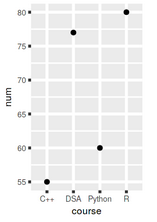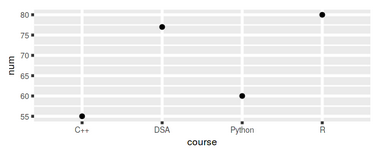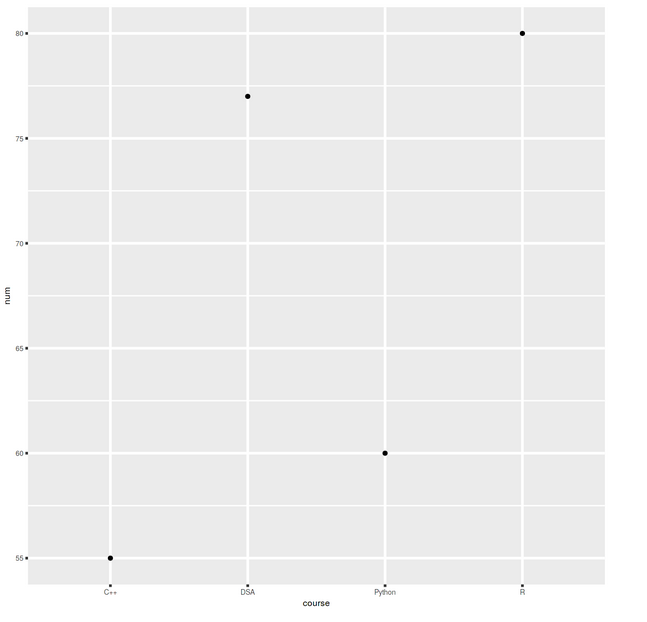# How to resize a graph in ggplot2 in R?

• Last Updated : 28 Feb, 2022

In this article, we are going to see how to resize the graph in ggplot2 in the R programming language. To resize the graph we like to use option() methods in R.

option() method: It returns the height and weight of the specific graph

Syntax: option(weight, height)

## Resizing a graph in ggplot2

Here we are going to see three different examples, for this, we will create a dataframe and then set the width and height using option() methods and then plot the graph using ggplot2.

### Example 1:

Under this example, we are plotting a point plot with height 3 and width 2.

## R

 `library``(ggplot2) ` ` `  `# Create data for chart ` `val <-``data.frame``(course=``c``(``'DSA'``,``'C++'``,``'R'``,``'Python'``), ` `                 ``num=``c``(77,55,80,60)) ` `options``(repr.plot.width = 2, repr.plot.height =3) ` ` `  `# Basic Line ` `ggplot``(data=val, ``aes``(x=course, y=num, group=1)) +``geom_point``()+ ` `theme``(text = ``element_text``(size = 9),``element_line``(size =1))`

Output:### Example 2:

In this example, we have passed the width to be 5 and height to 2 to the option() function to resize the plot,

## R

 `library``(ggplot2) ` ` `  `# Create data for chart ` `val <-``data.frame``(course=``c``(``'DSA'``,``'C++'``,``'R'``,``'Python'``), ` `                 ``num=``c``(77,55,80,60)) ` `options``(repr.plot.width = 5, repr.plot.height =2) ` ` `  `# Basic Line ` `ggplot``(data=val, ``aes``(x=course, y=num, group=1)) +``geom_point``()+ ` `theme``(text = ``element_text``(size = 9),``element_line``(size =1))`

Output:### Example 3:

In this example, we have kept width to be 9 and height to 9  to resize the plot,

## R

 `library``(ggplot2) ` ` `  `# Create data for chart ` `val <-``data.frame``(course=``c``(``'DSA'``,``'C++'``,``'R'``,``'Python'``), ` `                 ``num=``c``(77,55,80,60)) ` `options``(repr.plot.width =9, repr.plot.height =9) ` ` `  `# Basic Line ` `ggplot``(data=val, ``aes``(x=course, y=num, group=1)) +``geom_point``()+ ` `theme``(text = ``element_text``(size = 9),``element_line``(size =1))`

Output:My Personal Notes arrow_drop_up
Recommended Articles
Page :